In a live walk-through on April 10 at the Davis R-Users Group, I gave a brief presentation motivating this topic. This post expands and cleans up the code from that talk. If you just want the code: download the .R file.

Updated 2015-04-28

The point of this post isn’t the statistics of mixed models. For that, learning the experimental design and statistics behind modern mixed models, I recommend some relatively recent papers below. In short, Schielzeth & Nakagawa (2013) and Stroup (2015) provide especially good introduction for those coming from an ANOVA background.

When working with generalized, hierarchical designs, ask yourself three questions:

First (and maybe most important), “Do I really need these models?” Think carefully, and consult these references:

Second, “What is my design (in the language of mixed models)?”. Baayen et al focus on designs with crossed random effects (a strong suit of lme4), while Schielzeth & Nakagawa’s “Nested by Design” paper is a more general introduction. Finally, the Stroup paper provides a good entry point in ANOVA-speak 2. This could make or break the connection between an adviser that speaks only ANOVA, as is often the case for workers in crop and soil sciences, or experimental ecology and the GLMM that your unbalanced binomial regression demands.

Third, "How do I specify and fit this model in R. The references below may also help with design and interpretation, but are primarily hands-on. The most thorough is Pinheiro & Bates (2000). These four references also serve as a bibliography of sorts for this post 3.

### Focus of this post

Given these preliminaries, here I focus on three things:

• criticizing the model and fit
• (graphically) assessing parameter inference
• illustrate predictions

emphasizing

• working with frequentist library lme4 for (G)LMM in R
• visualization for: criticism, inference, prediction
• using the native plotting capabilities (to the extent possible)

three facets of visualization

• quick diagnostic (plots native to fitting packages)
• slower model criticism (also native)
• inference & prediction (generally lightweight external libraries / export capabilities)

Finally, I’ll mention that example code and vignettes in documented packages are great resources for learning these things (as is google ;). Try these commands:

??lme4
help(package='lme4')
methods(class='merMod')
vignette(package='lme4')

Packages:

library(lme4)
## Warning: package 'lme4' was built under R version 3.6.1
## Loading required package: Matrix
library(lattice) ## already loaded via namespace by lme4 -- may as well attach
#library(ggplot2) # recommend
#library(lsmeans) #recommend
#library(gridExtra) #recommend

Below, much of the commentary is included in # comments to the code blocks.

# LMM: greenhouse full factorial (RCBD)

• “Simple” greenhouse, linear mixed model
• Pattern and amount – cool question!
• balanced so perfect for traditional ANOVA
• 4 (!) treatments – full factorial blocked so 4-way interaction potential and hard to visualize (making a good test case here)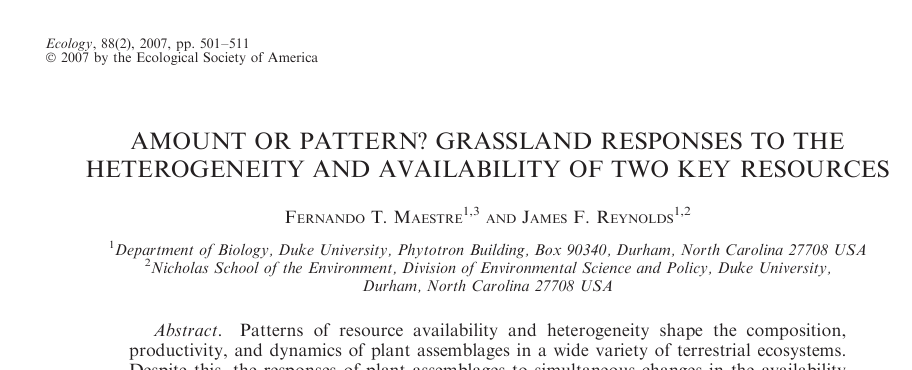Maestre title image

d <- read.delim("http://datadryad.org/bitstream/handle/10255/dryad.41984/Maestre_Ecol88.txt?sequence=1")
recode <- car::recode
## Registered S3 methods overwritten by 'car':
##   method                          from
##   influence.merMod                lme4
##   cooks.distance.influence.merMod lme4
##   dfbeta.influence.merMod         lme4
##   dfbetas.influence.merMod        lme4
d <- within(d, {
nutrient_hetero <- recode(factor(nh), "1='homogeneous'; 2='heterogeneous'")
nutrient_add <- recode(factor(na), "1='40 mg N'; 2='80 mg N'; 3='120 mg N'")
water_hetero <- recode(factor(wh), "1='homogeneous'; 2='pulse'")
water_add <- recode(factor(wa), "1='125 mL'; 2='250 mL';3='375 mL'")
block <- factor(block)
})
d$nutrient_add <- factor(d$nutrient_add, levels(d$nutrient_add)[c(2, 3, 1)]) ## centered? ctr <- function(v, ...) (v - mean(v, ...) ) #/ sd(v, ...) d <- within(d, { nhc <- ctr(nh) nac <- ctr(na) wac <- ctr(wa) whc <- ctr(wh) }) ## bt - total biomass ## we'd like to use model that includes varying sopes ## watering seems the most likely to have block-level differences ? m2 <- lmer(log(bt) ~ nutrient_add * nutrient_hetero * water_add * water_hetero + (water_hetero * water_add | block), data=d) ## boundary (singular) fit: see ?isSingular ## first diagnostic -- very high correlation is bad -- can't really justify complex RE ## structures you might like, if cause these type of fits! ## could see bad performance in resid plots too if you wanted VarCorr(m2) ## Groups Name Std.Dev. Corr ## block (Intercept) 0.031293 ## water_heteropulse 0.041218 -0.741 ## water_add250 mL 0.060201 -0.936 0.642 ## water_add375 mL 0.059746 -0.612 0.231 0.845 ## water_heteropulse:water_add250 mL 0.073590 0.937 -0.929 -0.852 ## water_heteropulse:water_add375 mL 0.073299 0.415 -0.110 -0.706 ## Residual 0.070228 ## ## ## ## ## ## -0.461 ## -0.969 0.290 ##  plot(m2, type = c("p", "smooth") , id = 0.05) # id outliers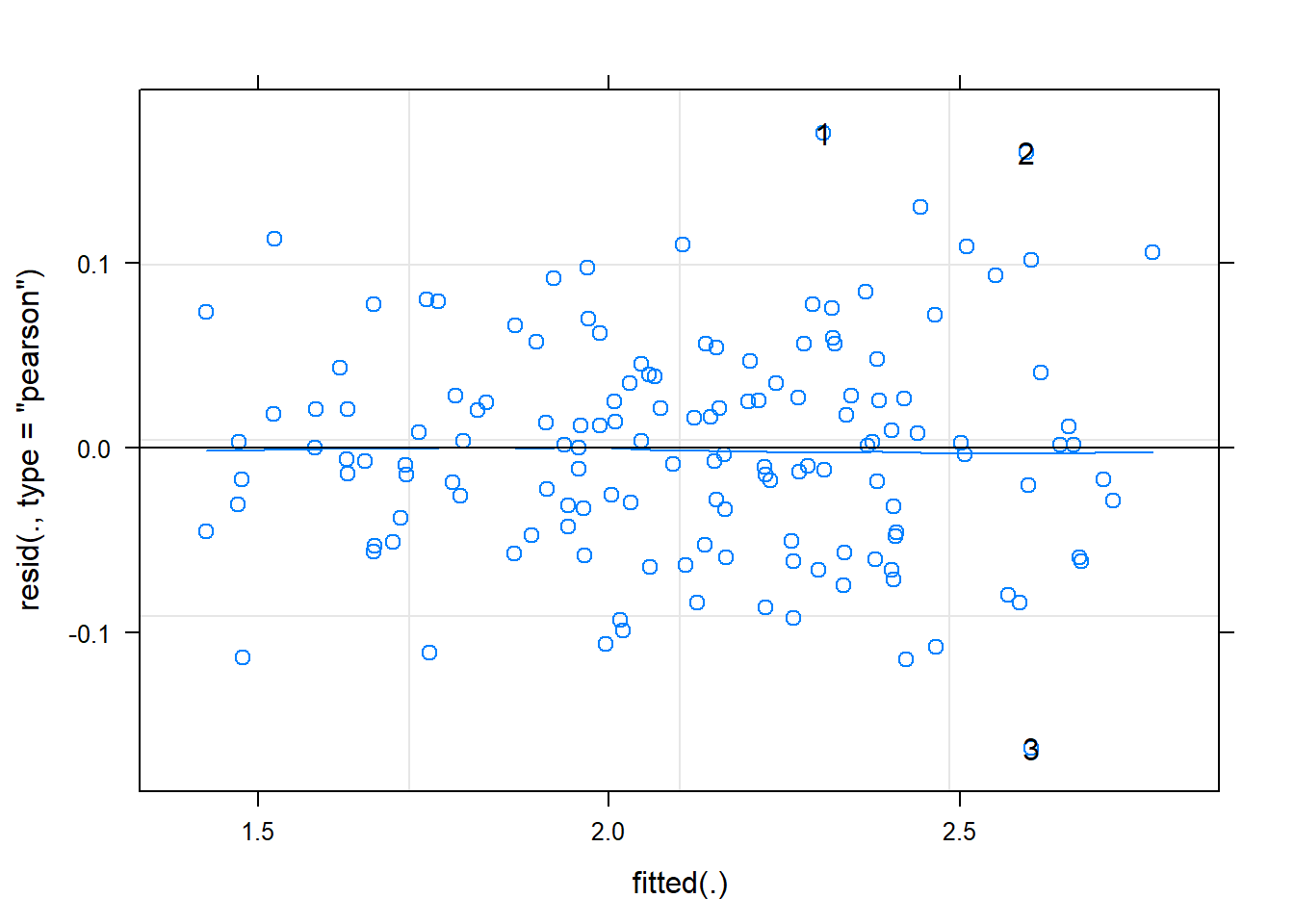plot(m2, sqrt(abs(resid(.))) ~ fitted(.), type=c('p', 'smooth'))## slight increase in resid w/ fitted plot(m2, resid(.) ~ fitted(.) | block, abline=c(h = 0), lty = 1, type = c("p", "smooth")) # per block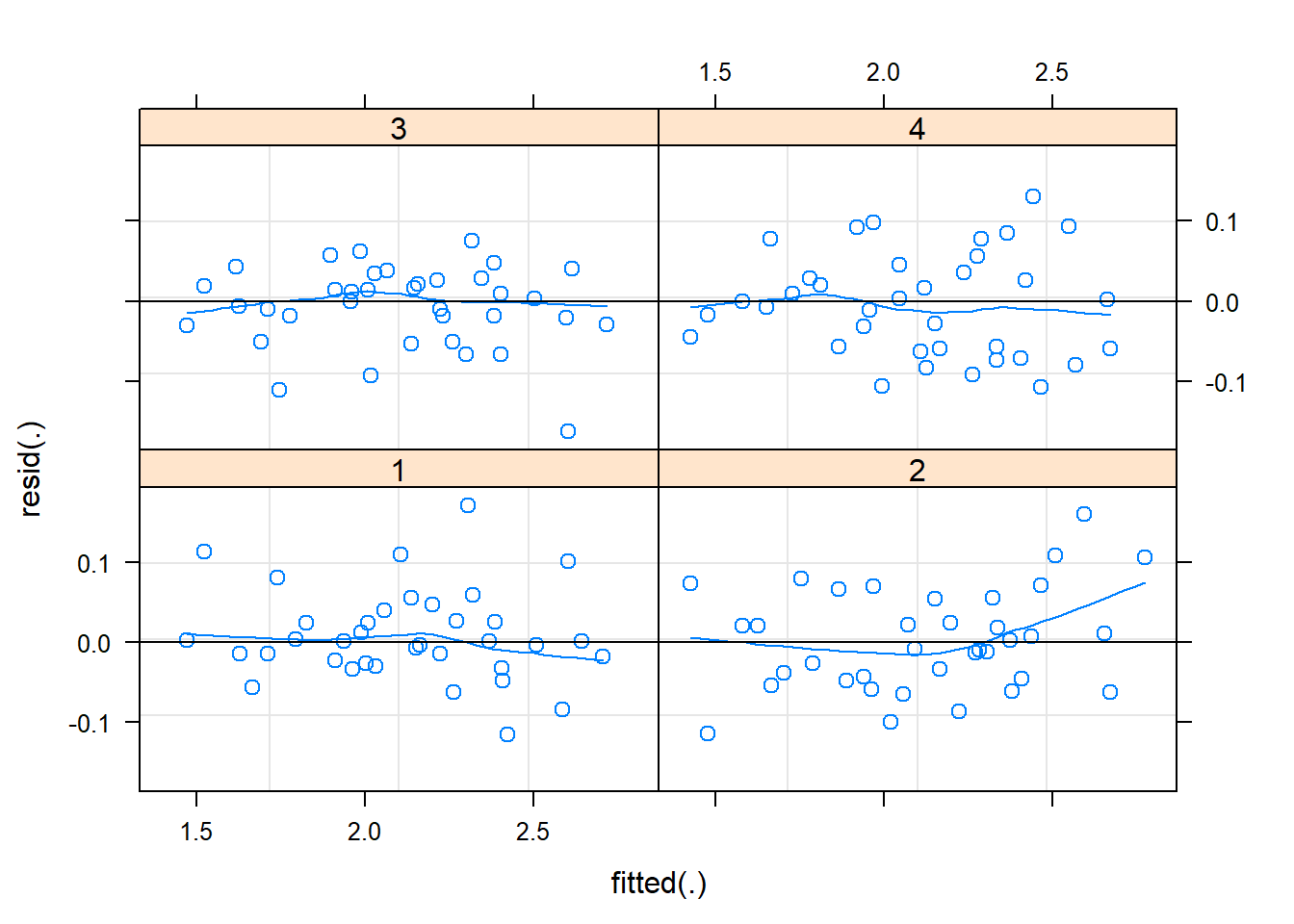plot(m2, sqrt(abs(resid(.))) ~ fitted(.) | block, type=c("p", "smooth")) # 2 blocks behave badly## block 4 is not great well behaved :( lattice::qqmath(m2)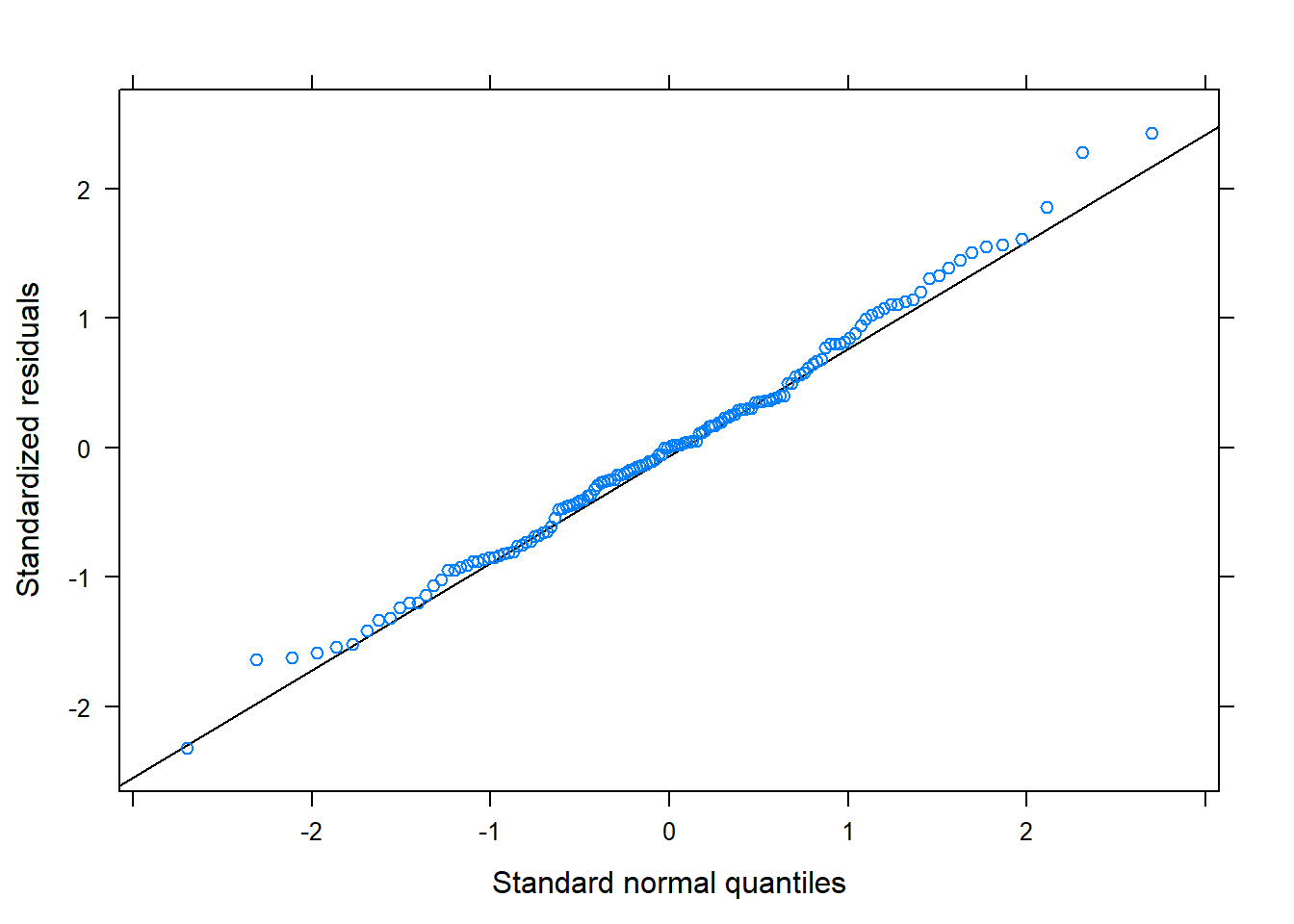## look at random effect lattice::dotplot(ranef(m2, condVar=TRUE)) ##$block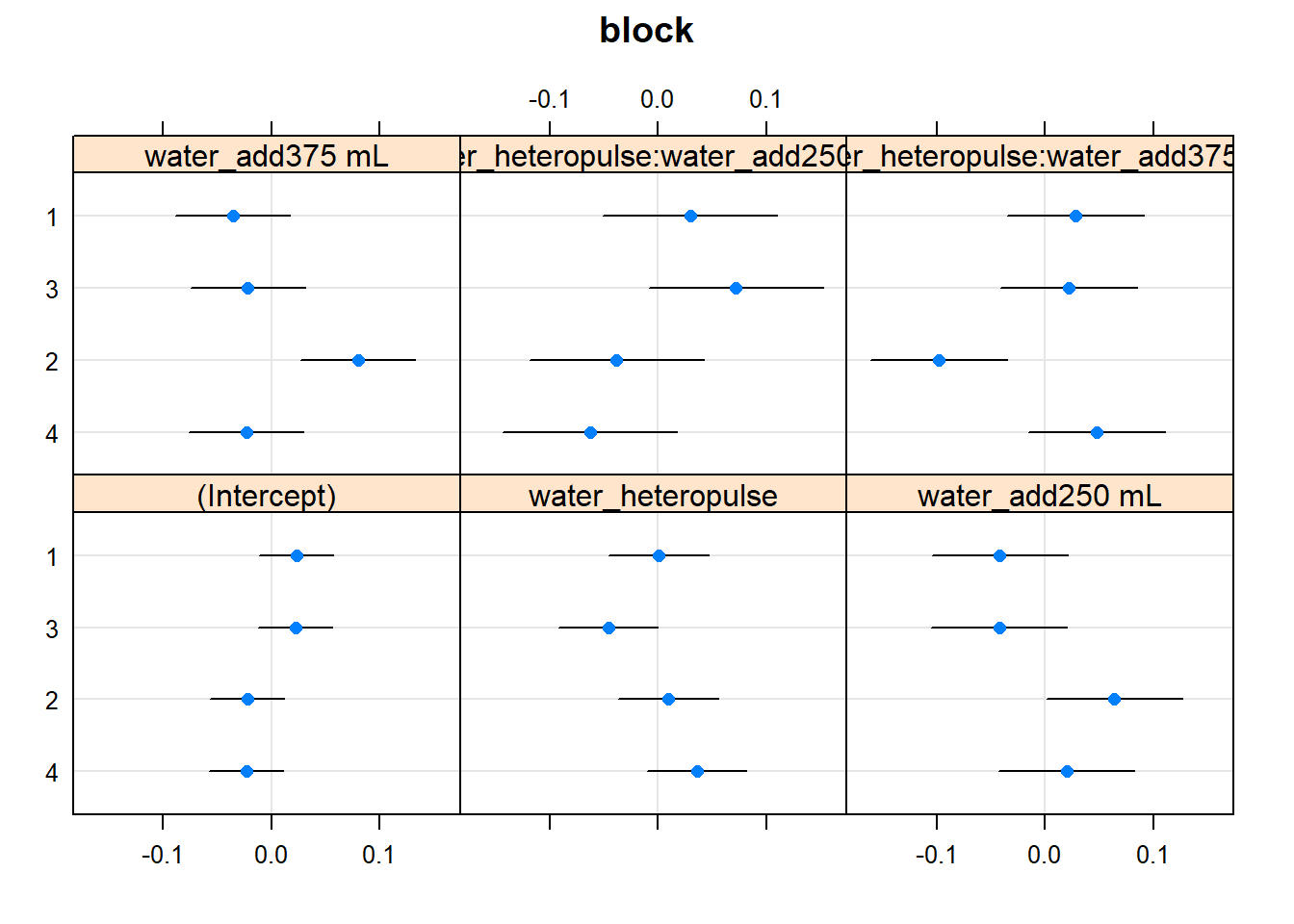##were we to have LOTS of RE, using lattice::qqmath for y axis spacing
##based on quantiles of standard normal -- is better to distinguish
##important few from "trivial many"

## want to look @ 4-way but profile diagnostics not working

## for illustrating these, I fit a model with fewer interactions
## use quick plot assess which fixef we can drop (in addition to the RE, unf)
## run drop1(m2) and drop 4-way and water_hetero:nutrient_add
nutrient_hetero *  water_add  * water_hetero  +
(1 | block),
data=d)

## Note: if you look at diagnostics for these, issues pointed out
## above seem even worse. there is a greater increase in resid w/ fitted
## several of the blocks are poorly behaved
## plot(m1, type=c("p", "smooth") , id=0.05) # id outliers
## plot(m1, sqrt(abs(resid(.))) ~ fitted(.), type=c('p', 'smooth'))
## plot(m1, resid(.) ~ fitted(.) | block, abline=c(h=0), lty=1,  type=c("p", "smooth")) # per block
## plot(m1,  sqrt(abs(resid(.))) ~ fitted(.) | block,     type=c("p", "smooth")) # 2 blocks behave  badly
## lattice::qqmath(m1)
## lattice::dotplot(ranef(m1, condVar=TRUE))
## to get more informative diagnostics -- need to profile
system.time(m1.prall <- profile(m1))
##    user  system elapsed
##    1.72    0.00    1.72
system.time(m1.prre <- profile(m1, which='theta_', maxmult=5)) ## lower maxmult to avoid step error
##    user  system elapsed
##    0.27    0.00    0.26
lattice::xyplot(m1.prall)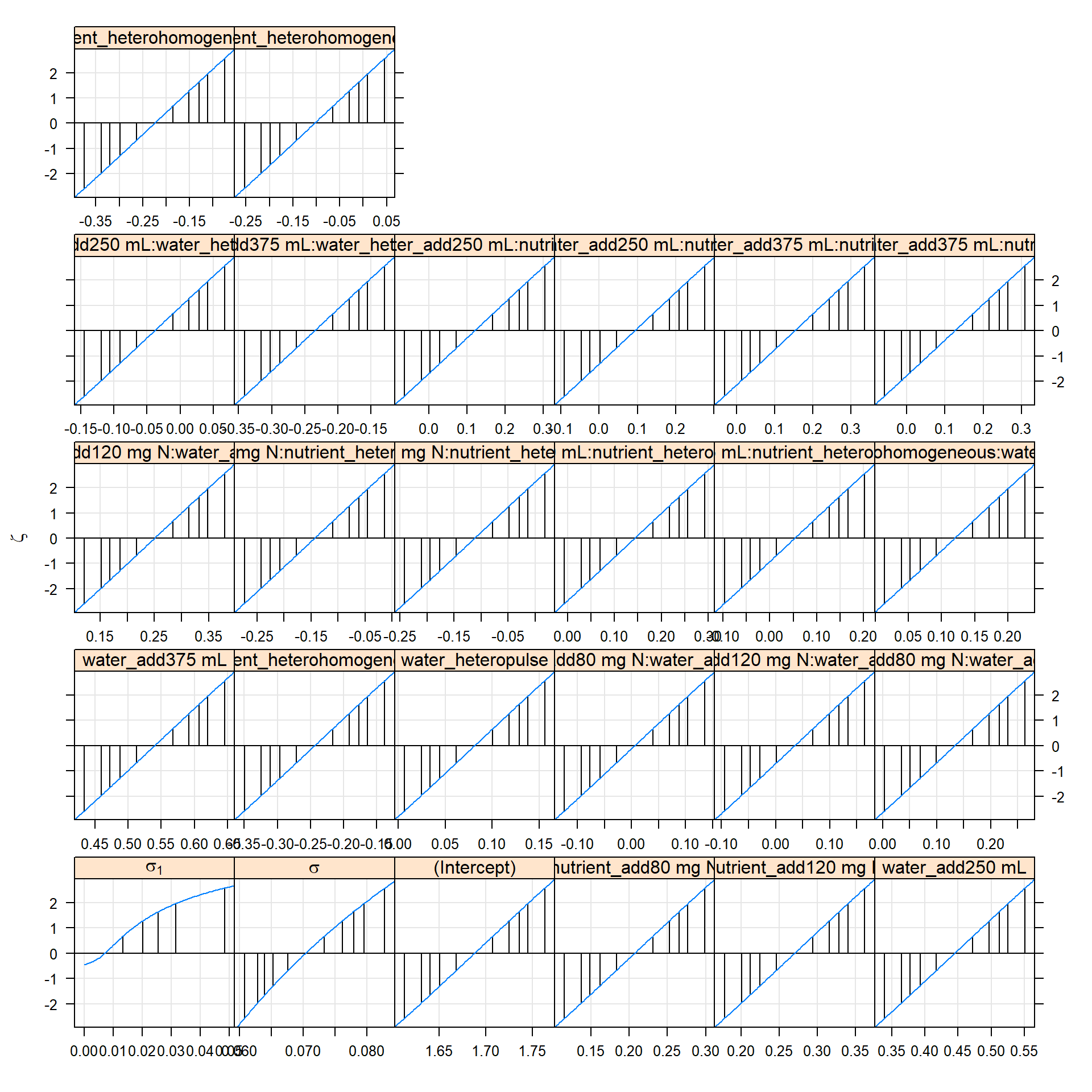# lattice::xyplot(m1.prre) # for only re
# the 'profile zeta plot' linear indicates a quadratic
# likelihood profile, and so Wald approximations for CI will work well
# random effects parameters on a standard deviation (or correlation)
# scale

lattice::densityplot(m1.prall)# the 'profile density plot'
# approximate probability density functions of the parameters
# -- distros underlying profile confidence intervals
# linear zeta plot <==> gaussian densities
lattice::splom(m1.prre)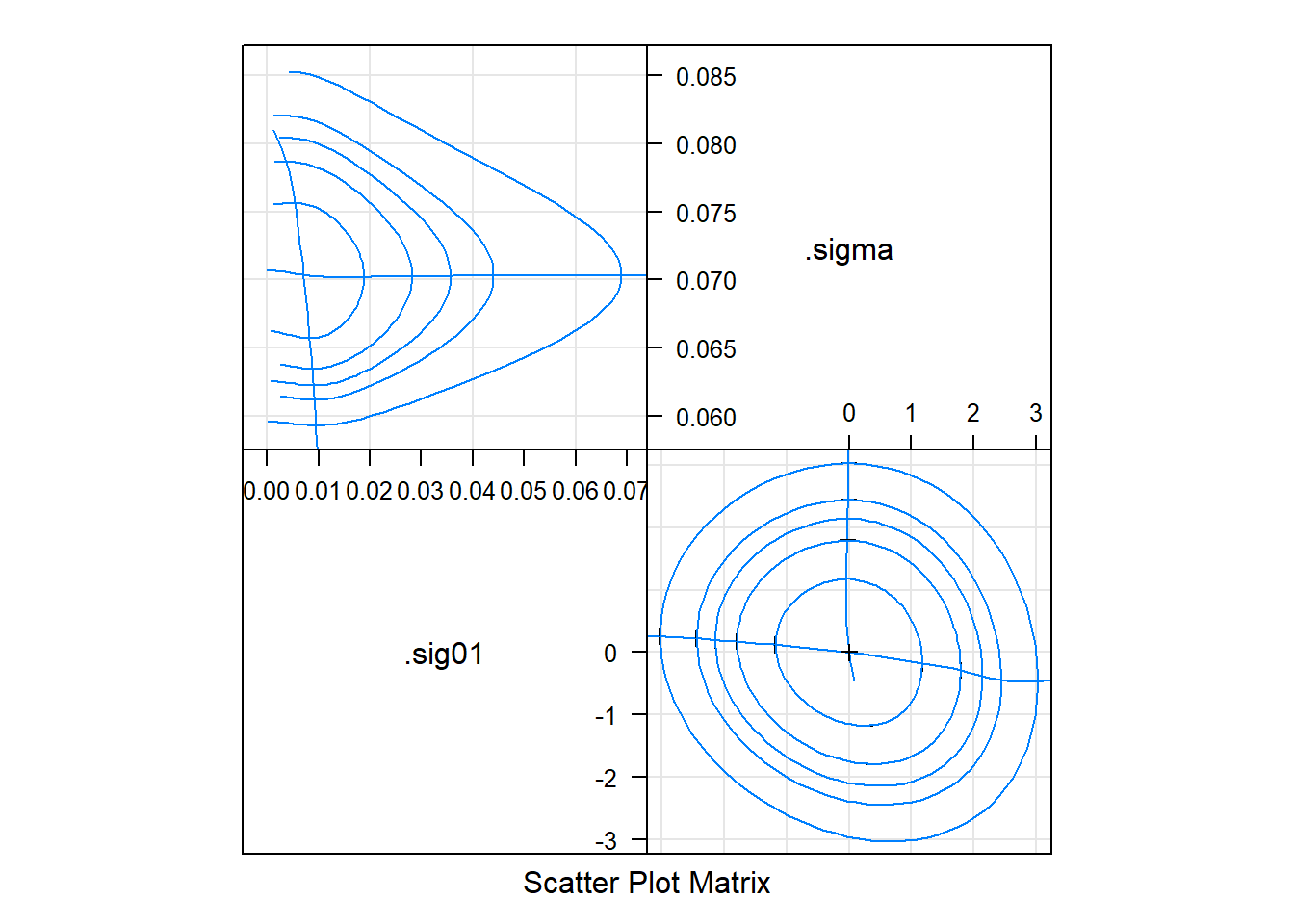# the 'profile pairs plot'
# bivariate confidence regions based on profile
# 'profile traces' -- cross hairs -- are conditional estimates  of one parameter given the other
# above-diag: estimated scale
# below-diag: 'zeta' scale, some sense, the best possible set of single-parameter transformations for assessing the contours"
# we just look at RE here

## Implications – lsmeans

Understanding a complex model with high-order interactions is tough. The best tool is plotting, but quickly visualizing such models is not easy with flexible plotting libraries.

Fortunately, lsmeans, although fairly inflexible in general, has plotting capabilities designed just for this purpose. Here’s a demo with the full (4-way interacting) model

## look at consequences
if(require(lsmeans)){
## here for 4-way interaction model (m2) -- mostly to see how can quickly plot response
## at factor levels in complex models
## relatively easy to plot mean prediction on response scale account for complex interaction
}
## Loading required package: lsmeans
## Warning in library(package, lib.loc = lib.loc, character.only = TRUE,
## logical.return = TRUE, : there is no package called 'lsmeans'
if(require(lsmeans)){
## can also plot quickly mean estimates plus (Wald) confidence intervals
## not that it's possible to do finite size correction in lsmeans (but only for linear models)
## steps take a min w/ lots of RE for some reason
plot(m2.lsm, layout =c(2, 3), horizontal=TRUE)
}
## Loading required package: lsmeans
## Warning in library(package, lib.loc = lib.loc, character.only = TRUE,
## logical.return = TRUE, : there is no package called 'lsmeans'
    ## for the simpler

## overall pattern doesn't differ too much from m1
plot(m1.lsm, layout =c(2, 3), horizontal=TRUE)

# Confidence intervals

## quick & dirty

## OK -- back to the full model
## if residuals looking OK -- fastest way to get a quick look at coefficient uncertainty
## I'll make it a function for later use

quick_CI_df <- function(model, drop_intercept = TRUE,...) {
## coef table using WALD CIs for a merMod
ci_dat <- confint(model, method='Wald', ...)
ci_dat <- cbind(ci_dat, mean=rowMeans(ci_dat))
ci_df <- data.frame(coef=row.names(ci_dat), ci_dat)
names(ci_df)[2:3] <- c('lwr', 'upr')
coef_colon <-   gregexpr("\\:", ci_df$coef) coef_order <- sapply(coef_colon, function(x) { if(x == -1) return(0) else return(length(x)) }) ci_df$coef <- reorder(ci_df$coef, coef_order) if (drop_intercept) return(subset(ci_df, coef != '(Intercept)')) ci_df } m1_wald_ci <- quick_CI_df(m2) lattice::dotplot(coef ~ mean, m1_wald_ci, panel = function(x, y) { panel.xyplot(x, y, pch=19) panel.segments(m1_wald_ci$lwr, y, m1_wald_ci$upr, y) panel.abline(v=0, lty=2) })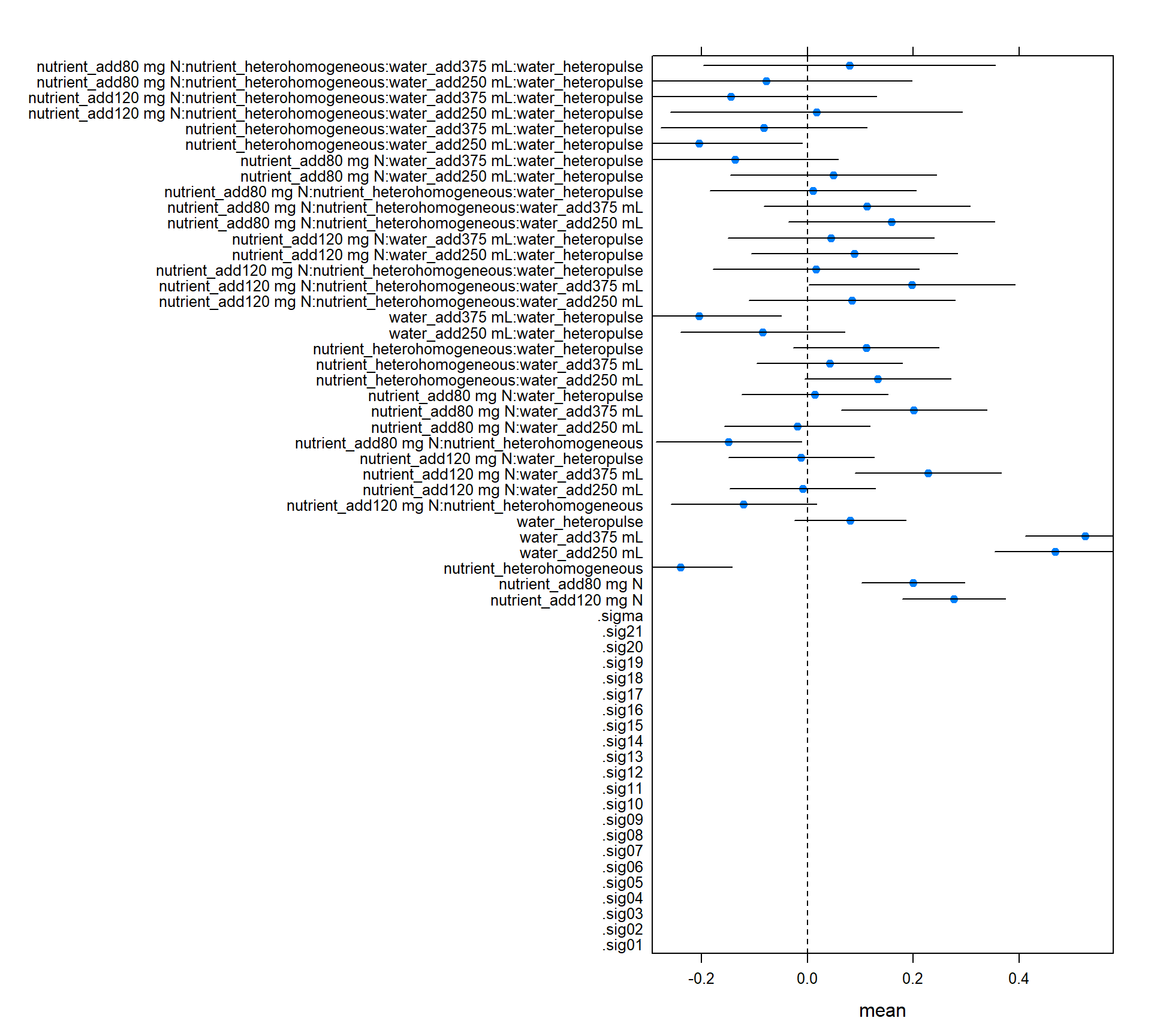## Confidence Intervals – rolling your own For more control than the simple plot above, you could use any of a variety of packages, e.g. coefplot2, arm::coefplot, here we just use builtin lme4::confint to build a dataframe. I make a dataframe with potentially several different types of confidence intervals: Wald (assume asymptotic multivariate normality of likelihood), profile, and bootstrap. For the random effects, the output of bootstrap and profile methods have different names – as you can see in the plot below. The Wald method only estimates CIs for fixed effects. compare_CI_df <- function(modprof, model, BOOT=FALSE, ...) { ci <- confint(model, method='Wald') cip <- confint(modprof) if(BOOT) { cib <- confint(model, method="boot", ...) cib_dat <- data.frame(cib, parameter=row.names(cib), type='Boot') names(cib_dat)[1:2] <- colnames(cib) } ci_dat <- data.frame(ci, parameter=row.names(ci), type='Wald') names(ci_dat)[1:2] <- colnames(ci) cip_dat <- data.frame(cip, parameter=row.names(cip), type='Prof') names(cip_dat)[1:2] <- colnames(cip) if (!BOOT) { ci_all_dat <- rbind(ci_dat, cip_dat) } else { ci_all_dat <- rbind(ci_dat, cip_dat, cib_dat) } fe_dat <- data.frame(parameter=names(fixef(model)), value=fixef(model)) list(mean=fe_dat, bounds=ci_all_dat) } if(require(ggplot2)) { m1_ci <- compare_CI_df(m1.prall, m1, BOOT=TRUE, nsim=100) m1_mean <- m1_ci[['mean']] m1_bnd <- m1_ci[['bounds']] ggplot(m1_bnd[m1_bnd$parameter != '(Intercept)', ]) +
geom_linerange(aes(parameter, ymax=97.5 %, ymin=2.5 %, color=type),
position=position_dodge(w=.5), size=1.5) +
geom_hline(yintercept=0, linetype=2) +
coord_flip() + theme_minimal() +
geom_point(aes(parameter, value), size=3, shape=3,
data=m1_mean[m1_mean$parameter != '(Intercept)', ]) } else { lattice::dotplot(parameter ~ c(2.5 %, 97.5 %) | type , m1_bnd, abline=c(v=0, lty=2)) } ## Loading required package: ggplot2 ## Computing bootstrap confidence intervals ... ## ## 38 message(s): boundary (singular) fit: see ?isSingular ## 1 warning(s): Model failed to converge with max|grad| = 0.00445866 (tol = 0.002, component 1) ## Warning: Removed 2 rows containing missing values (geom_linerange).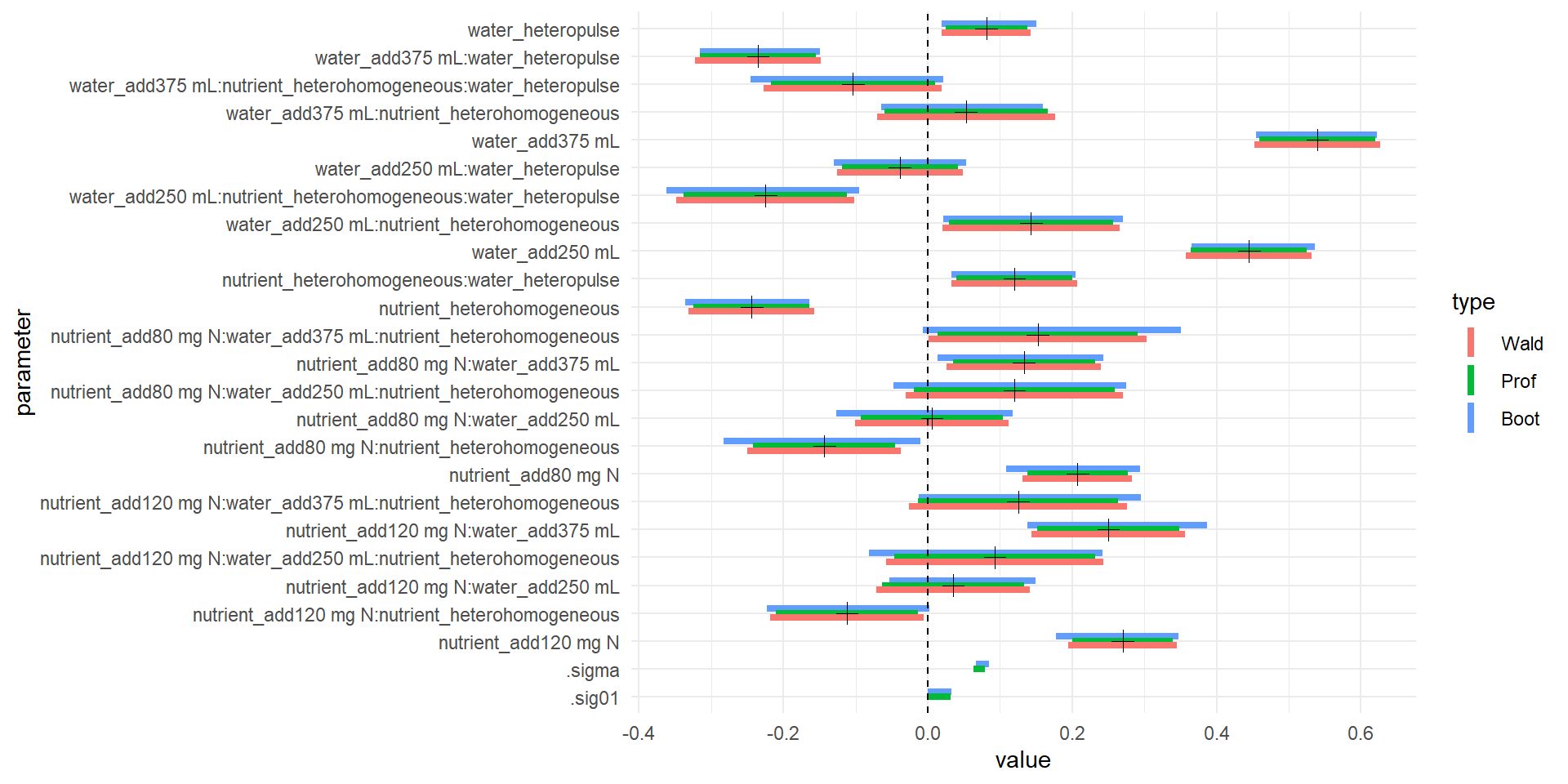## strong effect of nutrient homogeneity ## effect of adding lots of water ## nutrient has some effect ## Pulling data out of models with broom or ggplot2 Method ggplot2::fortify adds fitted and resid to data frame. New-ish CRAN package broom generalizes this (and more), really aiming provide an interface for ‘tidy’ (i.e., easy to plot)representations of model objects from many R functions (including packages and base R). The broom method augment really generalized version of fortify and is recommended instead if(require(ggplot2)) { d_fort <- ggplot2::fortify(m1, d) ## adds .fortify, scresid, .resid ##head(d_fort) } if(require(broom)) { glm1 <- glance(m1) # model summary tdm1 <- tidy(m1, effects='fixed') #model coefficient summary augm1 <- augment(m1, d, se.fit=TRUE, type.predict='response') # model data with fits ## head(glm1) ## head(tdm1) ## head(augm1) } ## Loading required package: broom Unfortunately, the predict and confint methods for merMod aren’t yet integrated to broom or ggplot (or my code above for confidence intervals could be much cleaner at the slight cost of an additional package dependency). ## GLMM: Lizard mate choice Mechanisms of speciation – cool question! • mate compatibility trails • western North American skinks (Plestiodon skiltonianus spp) • Richmond, J. Q., E. L. Jockusch, and A. M. Latimer 2010. Mechanical reproductive isolation facilitates parallel speciation in western North American scincid lizards. American Naturalist Repeated measure of ind crossed with multiple trials, binary outcome d2 <- read.delim("http://datadryad.org/bitstream/handle/10255/dryad.33579/Richmond%20et%20al%202011%20Datafile.txt?sequence=1") names(d2) <- gsub('\\.', '', names(d2)) # rescale geo dist, sizediff d2 <- within(d2, { geodist_scl <- (GeoDist - mean(GeoDist)) / var(GeoDist) gendist_scl <- (GenDist - mean(GenDist)) sizediff_scl2 <- ((SizeDiff - mean(SizeDiff)) / var(SizeDiff))^2 Series <- factor(Series) Female <- factor(Female) Male <- factor(Male) }) ## cop - copulation system.time(gm0 <- glmer(cop ~ sizediff_scl2 + (1 | Series) + (1 | Male) + (1 | Female) , data=d2, family='binomial')) ## boundary (singular) fit: see ?isSingular ## user system elapsed ## 0.41 0.00 0.41 system.time(gm1 <- glmer(cop ~ gendist_scl + geodist_scl + sizediff_scl2 + (1 | Series) + (1 | Male) + (1 | Female) , data=d2, family='binomial')) ## boundary (singular) fit: see ?isSingular ## user system elapsed ## 1.03 0.00 1.03 anova(gm1, gm0) # no need for non-size predictors ## Data: d2 ## Models: ## gm0: cop ~ sizediff_scl2 + (1 | Series) + (1 | Male) + (1 | Female) ## gm1: cop ~ gendist_scl + geodist_scl + sizediff_scl2 + (1 | Series) + ## gm1: (1 | Male) + (1 | Female) ## Df AIC BIC logLik deviance Chisq Chi Df Pr(>Chisq) ## gm0 5 159.34 176.47 -74.673 149.34 ## gm1 7 162.92 186.90 -74.460 148.92 0.4251 2 0.8085 plot(gm0, type=c("p"), id = 0.05 )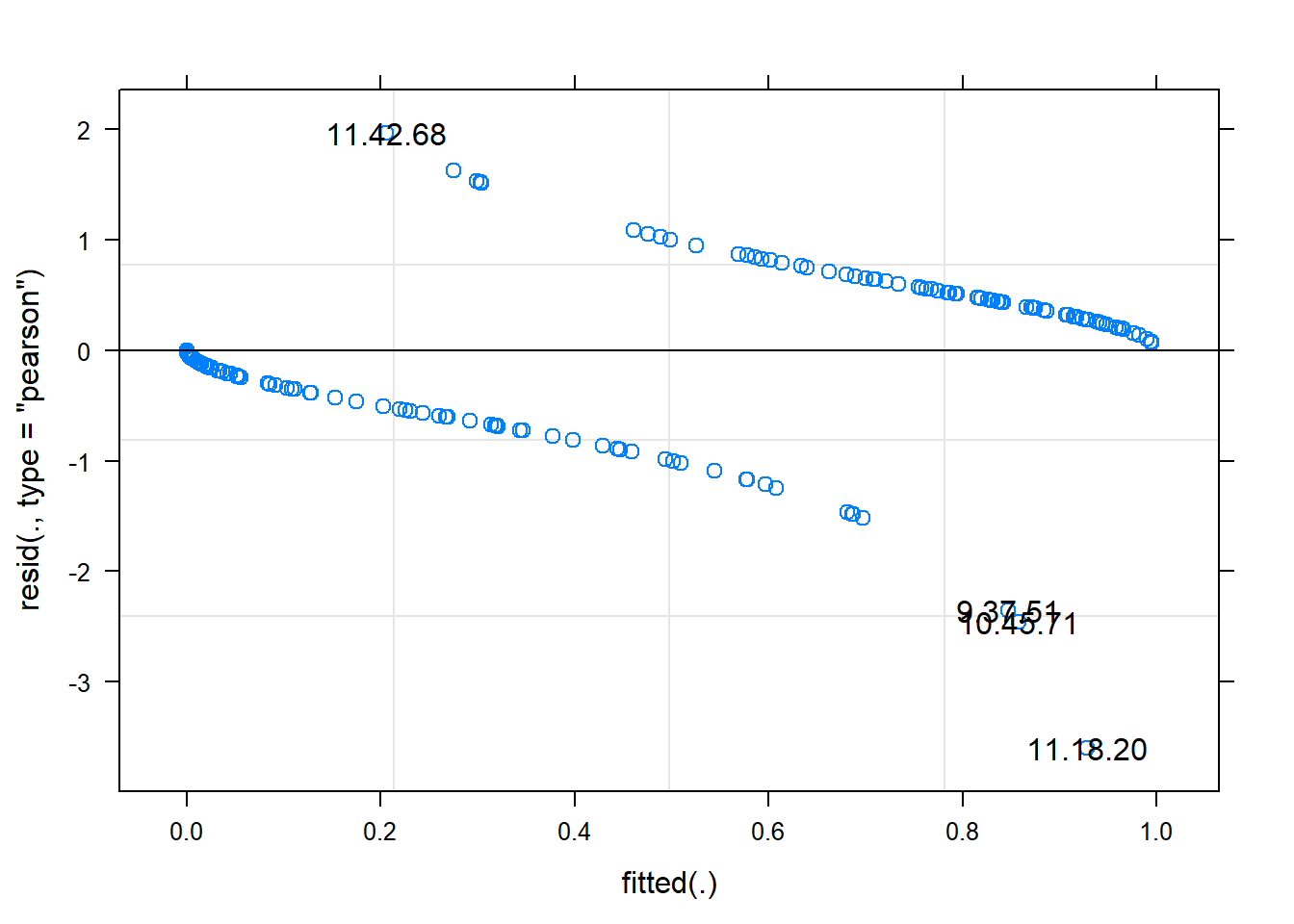## non useful visually but with id = 0.05 gives sense of outlier ## see ?plot.merMod for explanation of test run ## it's more useful to plot residuals in GLM logistic combined across grouping factors ## or binned fitted values plot(gm0, factor(Trial) ~ resid(., type='pearson'), abline=c(v=0), lty=2)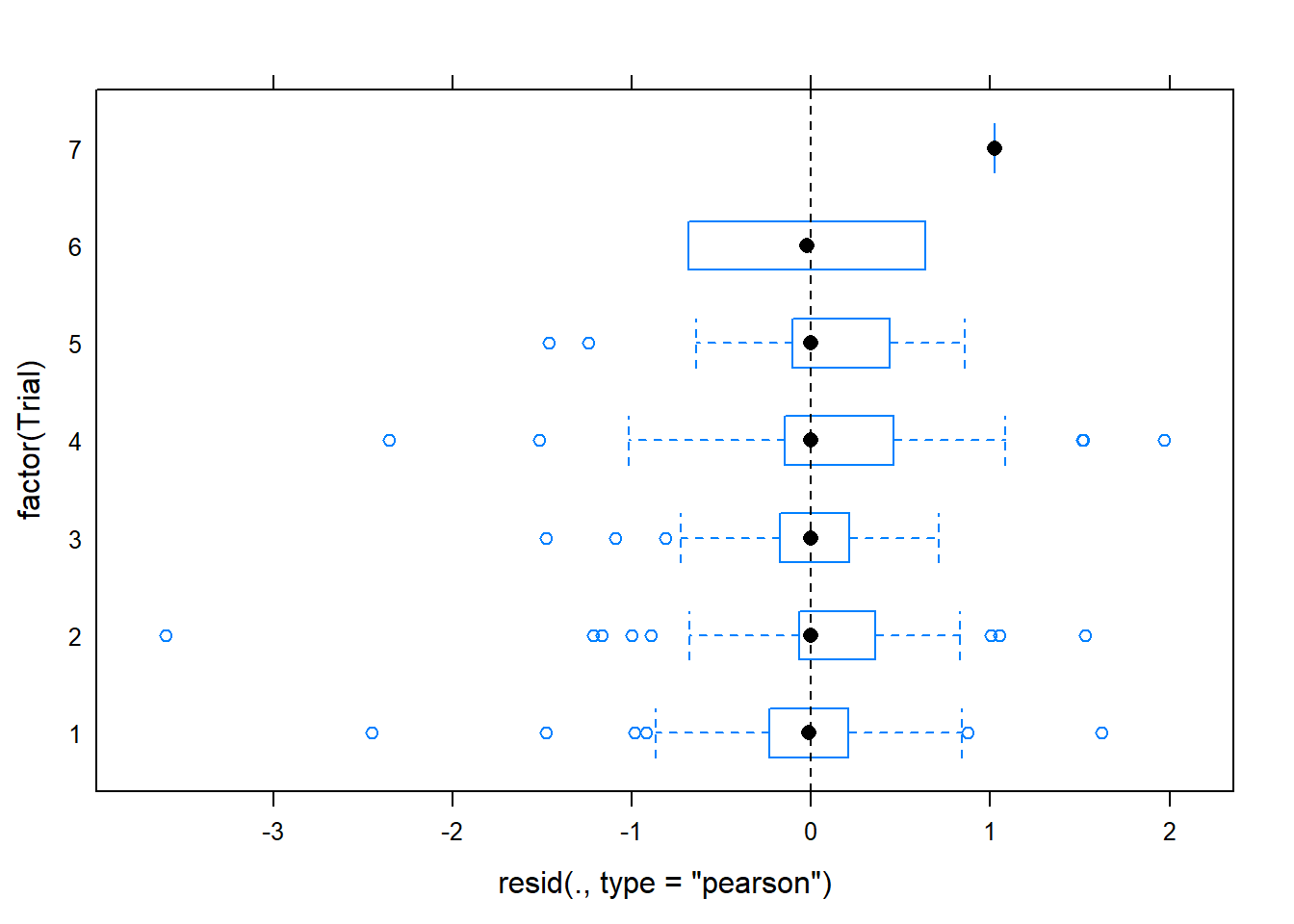plot(gm0, factor(Series) ~ resid(., type='pearson'), abline=c(v=0), lty=2)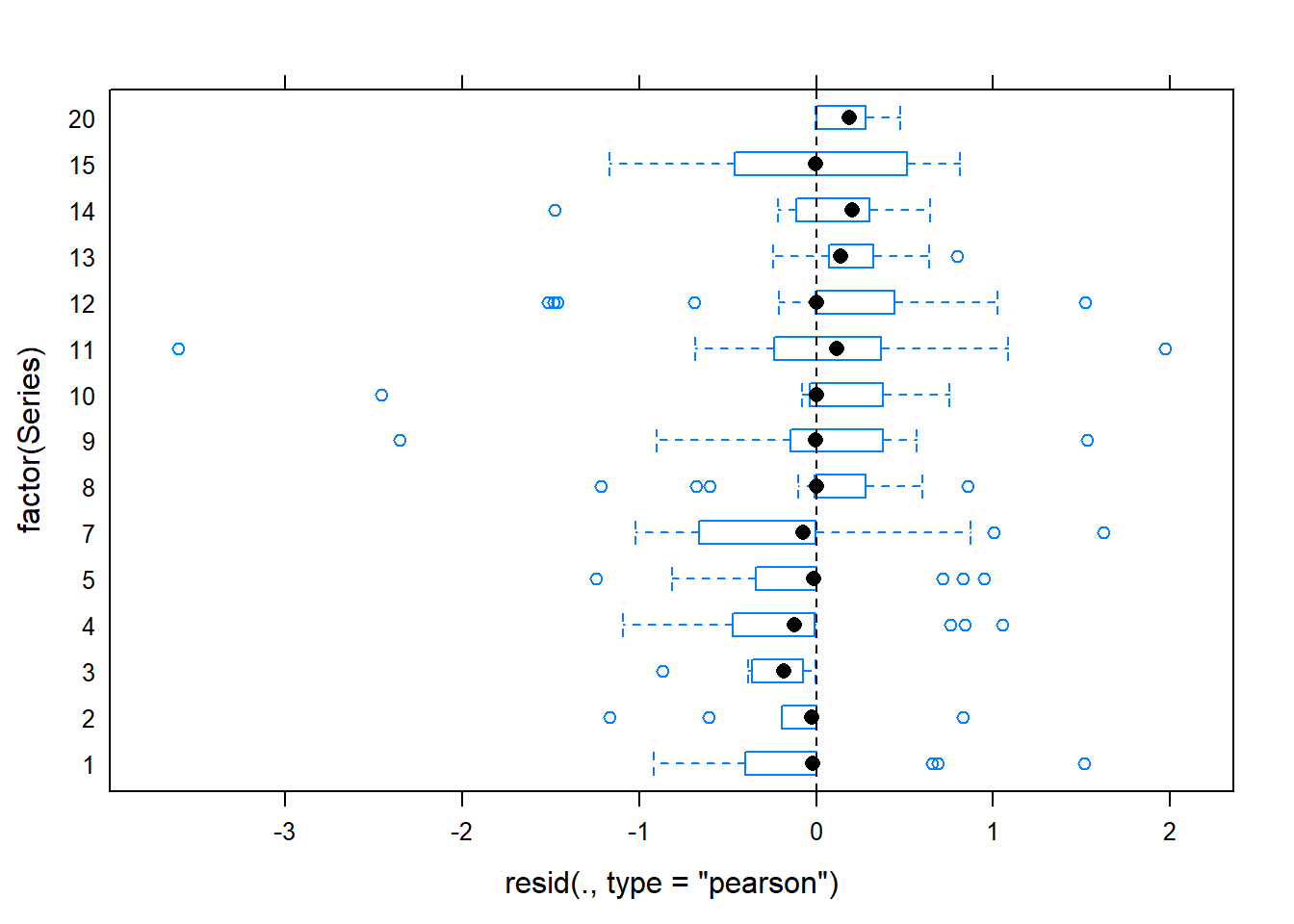## better than default eh ## second plot suggests some issue with series, indicating we may want to ## fit a model accounting for differences between trial series ## (in fact, Richmond et al did fit such a model) ## you can see the outliers here too with ## plot(gm0, resid(., type='pearson') ~ fitted(.) | factor(Series), id = 0.05) ## main thing on pearson or deviance residuals is they're mostly less than 2 ## (as assessed in first plot above -- second is the sqrt ## we could later drop these outlier and see if they change if(require(gridExtra)) { do.call(gridExtra::grid.arrange, c(lattice::qqmath(ranef(gm0, condVar=TRUE)), list(nrow=1))) } else { lattice::qqmath(ranef(gm0, condVar=TRUE)) } ## Loading required package: gridExtra ## Warning: package 'gridExtra' was built under R version 3.6.1## useful if you have a lot of RE to draw attention to strong ones ## maybe latter == dot = sample size? ## no reason to retain RE on Male, but also drop Female ## no real justification, just very slow profiling for many RE gm0noindre <- glmer(cop ~ sizediff_scl2 + (1 | Series), data=d2, family='binomial') system.time(# gm0.prall <- profile(gm0noindre, devtol=1e-6) # see https://stat.ethz.ch/pipermail/r-sig-mixed-models/2014q3/022394.html ) ## user system elapsed ## 5.66 0.00 5.67 #system.time( # gm0_full.prall <- profile(gm0, devtol=1e-6) #) # user system elapsed #344.092 0.539 344.613 lattice::xyplot(logProf(gm0.prall))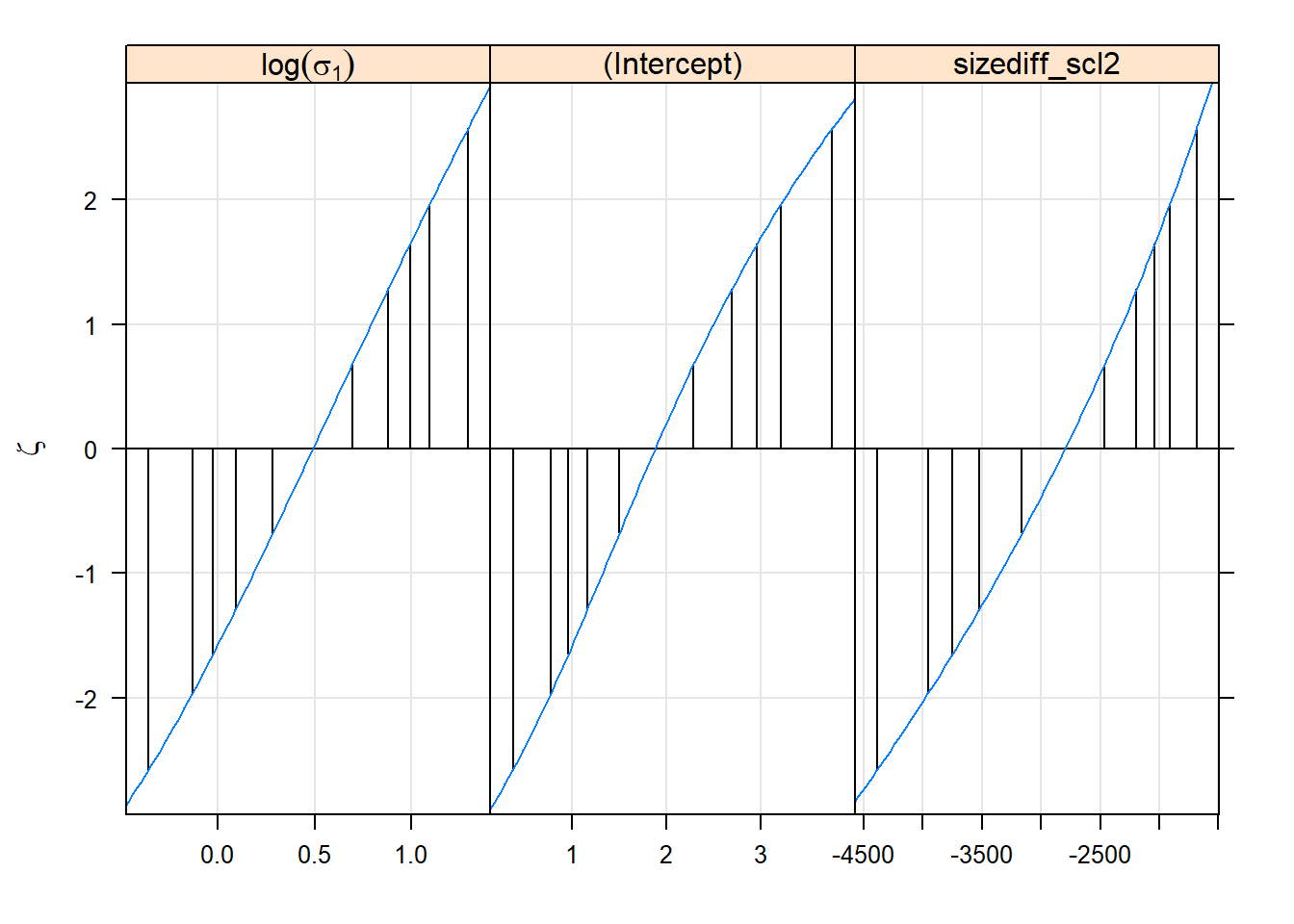lattice::densityplot(gm0.prall)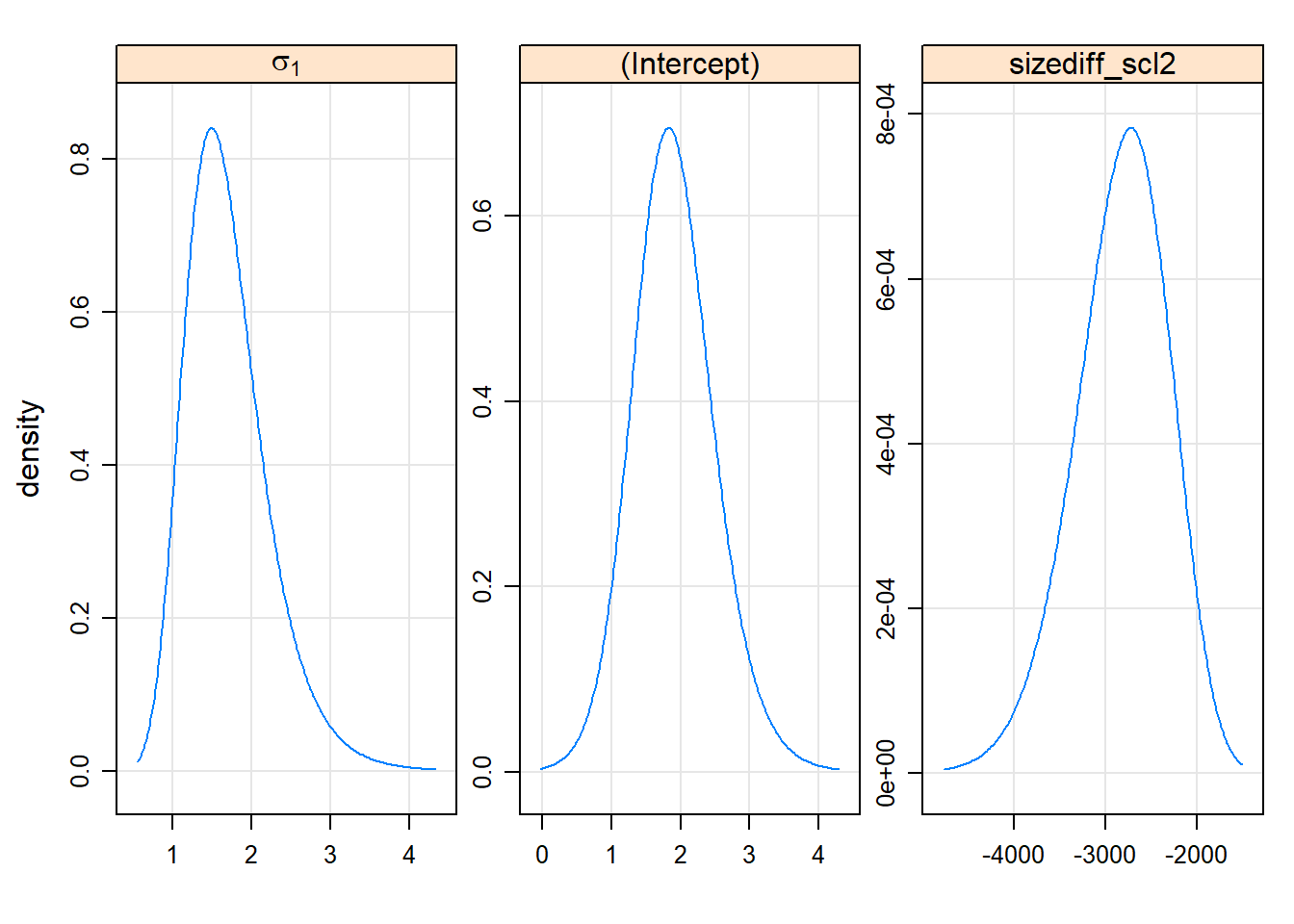lattice::splom(gm0.prall)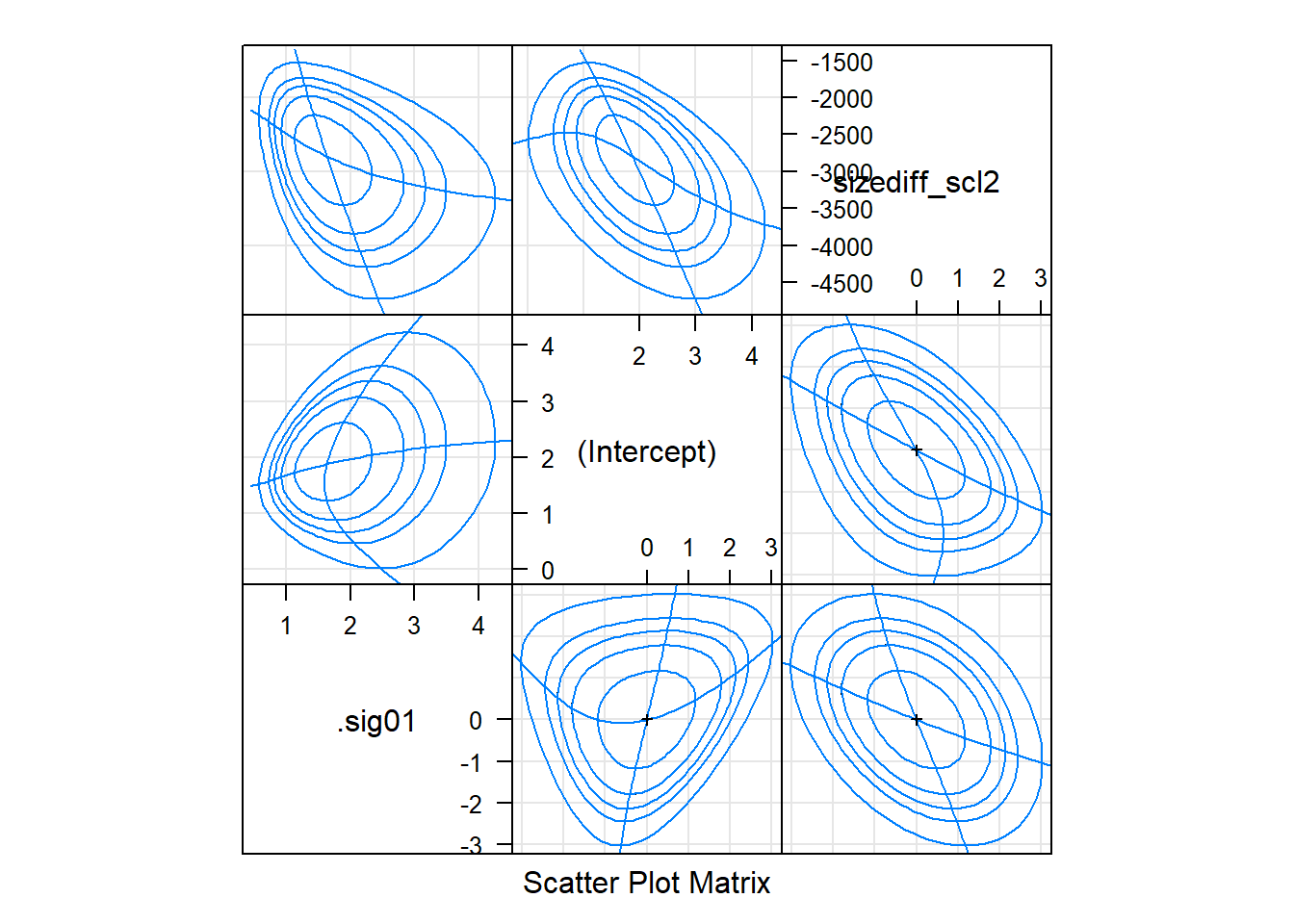# Confidence intervals ## if residuals looking OK -- fastest way to get a quick look at coefficient uncertainty ci_dat <- confint(gm0, method='Wald') ci_dat <- cbind(ci_dat, mean=rowMeans(ci_dat)) ci_df <- data.frame(coef=row.names(ci_dat), ci_dat) names(ci_df)[2:3] <- c('lwr', 'upr') lattice::dotplot(coef ~ mean, ci_df, panel = function(x, y) { panel.xyplot(x, y, pch=19) panel.segments(ci_df$lwr, y, ci_df$upr, y) panel.abline(v=0, lty=2) })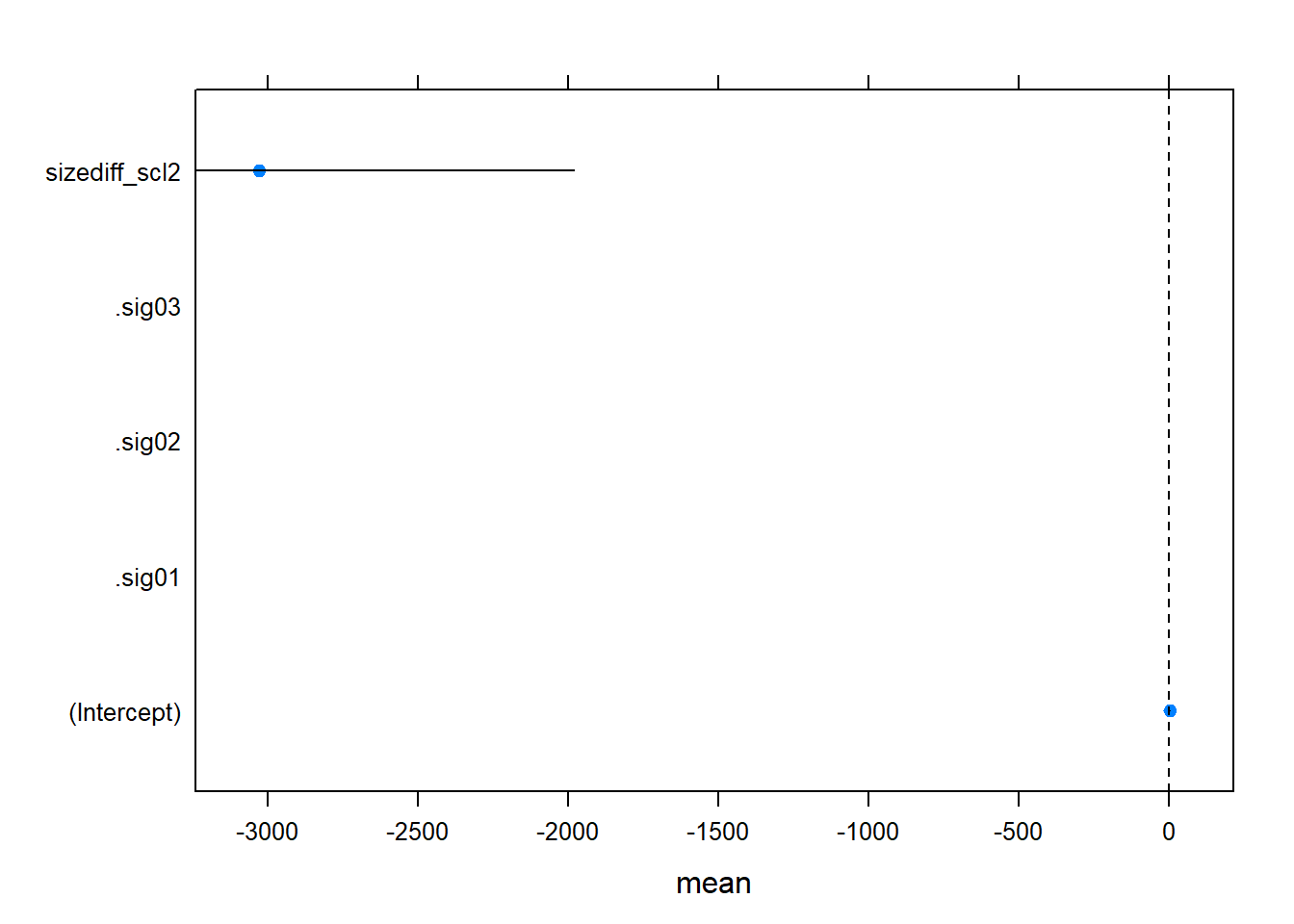Rolling your own again if(require(ggplot2)) { gm0_ci <- compare_CI_df(gm0.prall, gm0) gm0_mean <- gm0_ci[['mean']] gm0_bnd <- gm0_ci[['bounds']] g <- ggplot(gm0_bnd[gm0_bnd$parameter != '(Intercept)', ]) +
geom_linerange(aes(parameter, ymax=97.5 %, ymin=2.5 %, color=type),
position=position_dodge(w=.5), size=1.5) +
geom_hline(yintercept=0, linetype=2) +
coord_flip() + theme_minimal() +
geom_point(aes(parameter, value),
size=3, shape=3,
data=gm0_mean[gm0_mean$parameter != '(Intercept)', ]) g } else { lattice::dotplot(parameter ~ c(2.5 %, 97.5 %) | type , gm0_bnd) }Because the fixed effect parameter on size difference and the random effect variance are on such different scales, I make two plots if(require(ggplot2)) g + scale_y_log10()## quick predictions Again, the quickest way to visualize predictions is with lsmeans. Two things are different here: • to plot GLM predictions on a meaningful scale, you need to pass type = 'response' to the plot function. • for a quantitative predictor, the default will plot a single point at the mean of the predictor, to see prediction across the range, pass a list to the at argument • for more meaningful plots, we should label the plot y-axis with unscaled size differences (but I didn’t do this) • note that you can produce plots at various levels of interacting quantitative predictors using this technique if(require(lsmeans)) gm0_lsm <- lsmeans(gm0, ~ sizediff_scl2, at = list(sizediff_scl2 = seq(from=0, to = 1.5 * median(d2$sizediff_scl2), length.out = 10)))
## Loading required package: lsmeans
## Warning: package 'lsmeans' was built under R version 3.6.1
## Loading required package: emmeans
## Warning: package 'emmeans' was built under R version 3.6.1
## The 'lsmeans' package is now basically a front end for 'emmeans'.
## Users are encouraged to switch the rest of the way.
## convert old 'lsmeans' objects and scripts to work with 'emmeans'.
## Warning in vcov.merMod(object, correlation = FALSE): variance-covariance matrix computed from finite-difference Hessian is
## not positive definite or contains NA values: falling back to var-cov estimated from RX
plot(gm0_lsm, type = 'response', ylab = 'scaled size diff', xlab = 'probability of copulation')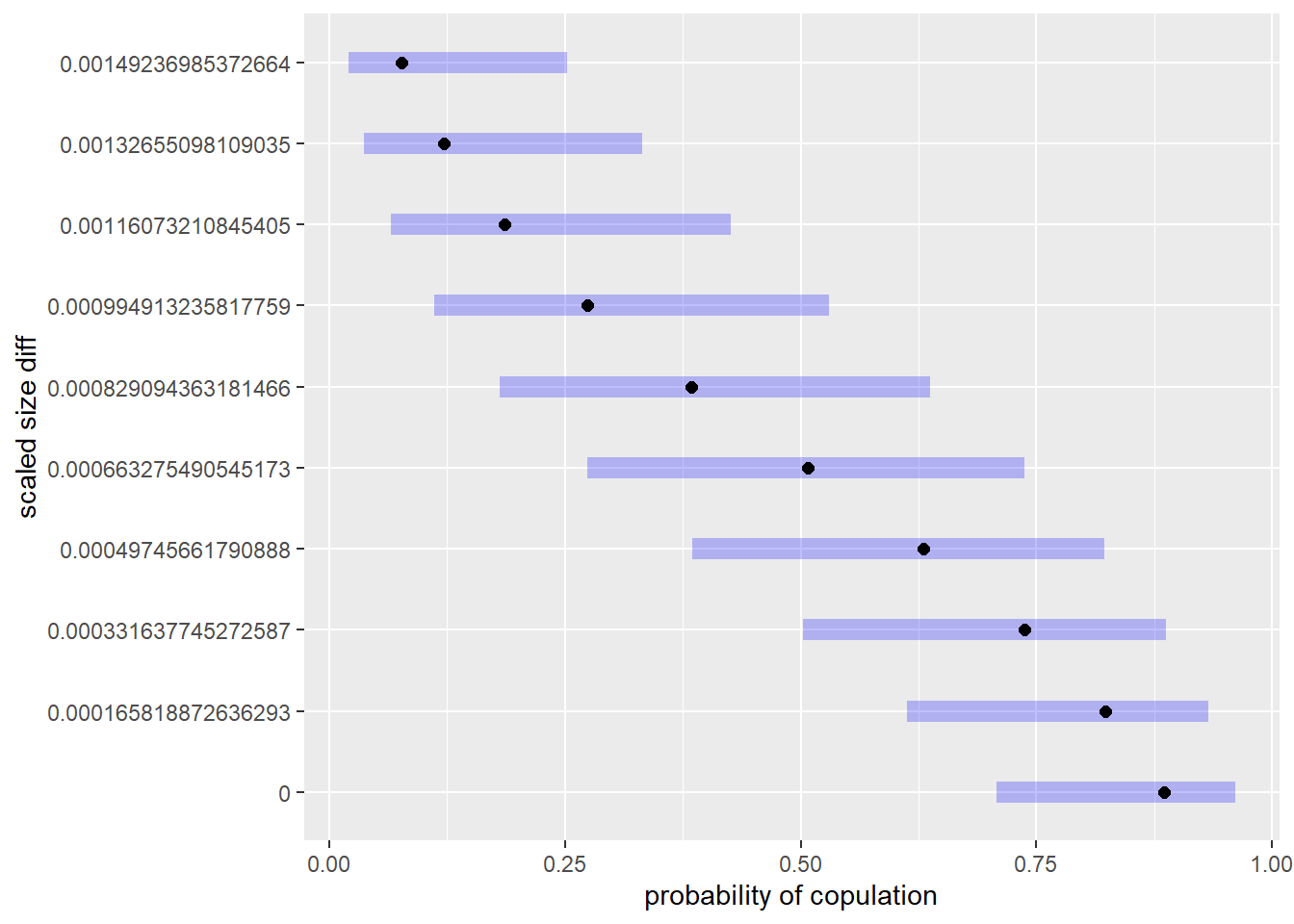## customized predictions

The quick plots using lsmeans are great, but they assume Wald confidence intervals and don’t account for random effects. To deal with the former, we need to bootstrap, which I won’t cover here, but the problem of random effects is easily examined using the re.form argument of predict.merMod in lme4. Here I look at predictions accounting for all,, none, or some of random effects fitted:

## predicted effect
d2$pred_overall_gen <- predict(gm0, type='response', re.form=NA) d2$pred_overall <- predict(gm0, type='response')
d2$pred_ind <- predict(gm0, type='response', re.form=~ (1|Female)) d2$pred_trial <- predict(gm0, type='response', re.form=~(1|Series))

## NB this changes row order from the fit!!!
d2_pred <- merge(d2, as.data.frame(table(Series=d2$Series))) if(require(ggplot2)) { g <- ggplot(d2_pred) + geom_point(aes(SizeDiff, cop), position=position_jitter(h=0.025, w=0)) + theme_minimal() + ylab("Probability copulation") g1 <- g + geom_line(aes(SizeDiff, pred_trial, color=Series)) g2 <- g + geom_line(aes(SizeDiff, pred_overall_gen), color='orange', size = 1.5) + geom_point(aes(SizeDiff, pred_ind, alpha=as.numeric(Female)), color = 'blue') + theme(legend.position = "none") gridExtra::grid.arrange(g1, g2, ncol=2) } else { plot(cop ~ SizeDiff, d2_pred, ylab="Probability copulation") points(pred_ind ~ SizeDiff, d2_pred, pch=19, cex=.5, col='darkgray') points(pred_overall_gen ~ SizeDiff, d2_pred, pch=19, cex=.5) }The plot on the left above shows predictions for various series of experimental trials, and the raw data. The plot on the right shows the overall fit (orange line – the grand mean with no random effects influencing the prediction), the predictions accounting just for random effects at the level of individual females (blue dots), and the raw data. ## could plot them all together -- code below gives some insight ## into the ## ggplot2 fortify method if (require(ggplot2)) { g + geom_point(aes(SizeDiff, plogis(.fitted)), color='darkgray', size=3, data=fortify(gm0, d2)) ## nb -- same as predict with all RE! with(fortify(gm0, d2), unique(pred_overall - plogis(.fitted))) } ## Checking model adequacy With lme4, you can also easily produce something akin to posterior-predictive checks, a Bayesian tool for model criticism. Using simulate on an lme4 produces a set of response data implied by the model. By examining summary statistics of this dataset, and comparing them to the actual data, you can examine how well, or poorly, the model fits. Note that these checks are less rigorous than something like cross-validation. See ?simulate.merMod for additional information. The upshot: these are relatively quick, and can provide a warning that your model isn’t capturing some aspect of the data. But, be aware there is controversy about their use for anything other than seeing how the model is capturing the data. Often, we’re interested in making more formal, frequentist inference. Though these checks are valuable, the p-like-values implied by them do not satisfy assumptions necessary for this kind of inference (i.e., don’t use them for model comparison or parameter inference). ## without RE set.seed(1141) gm0.sim_wo <- simulate(gm0, nsim=1000, re.form=NA) sims <- sapply(gm0.sim_wo, function(x) x) ones <- colSums(sims) zeros <- -colSums(sims-1) perc <- ones/nrow(d2) cvar <- apply(sims, 2, var) ## with RE gm0.sim <- simulate(gm0, 1000 ) sims <- sapply(gm0.sim, function(x) x) re_ones <- colSums(sims) re_zeros <- -colSums(sims-1) re_perc <- re_ones/nrow(d2) re_cvar <- apply(sims, 2, var) pp_comp <- function(dist, cmp_fn, data, ...) { hist(dist, col='orange', xlab='value', ...) abline(v=cmp_fn(data), lwd=4, col='blue', lty=2) abline(v=mean(dist), lwd=4) } op <- par(mfrow=c(2,4)) pp_comp(ones, function(x) sum(x$cop),
d2, main='ones - no RE', xlim=c(45, 110))
pp_comp(zeros, function(x) -sum(x$cop - 1), d2, main='zeros -no RE', xlim=c(120, 185)) pp_comp(perc, function(x) sum(x$cop)/nrow(d2),
d2, main='% cop - no RE', xlim=c(0, .8))
pp_comp(cvar, function(x) var(x$cop), d2, main='var - no RE') pp_comp(re_ones, function(x) sum(x$cop),
d2, main='ones - all RE', xlim=c(45, 110))
pp_comp(re_zeros, function(x) -sum(x$cop - 1), d2, main='zeros - all RE', xlim=c(120, 185)) pp_comp(re_perc, function(x) sum(x$cop)/nrow(d2),
d2, main='% cop - RE', xlim=c(0, .8))
pp_comp(re_cvar, function(x) var(x\$cop),
d2, main='var - all RE')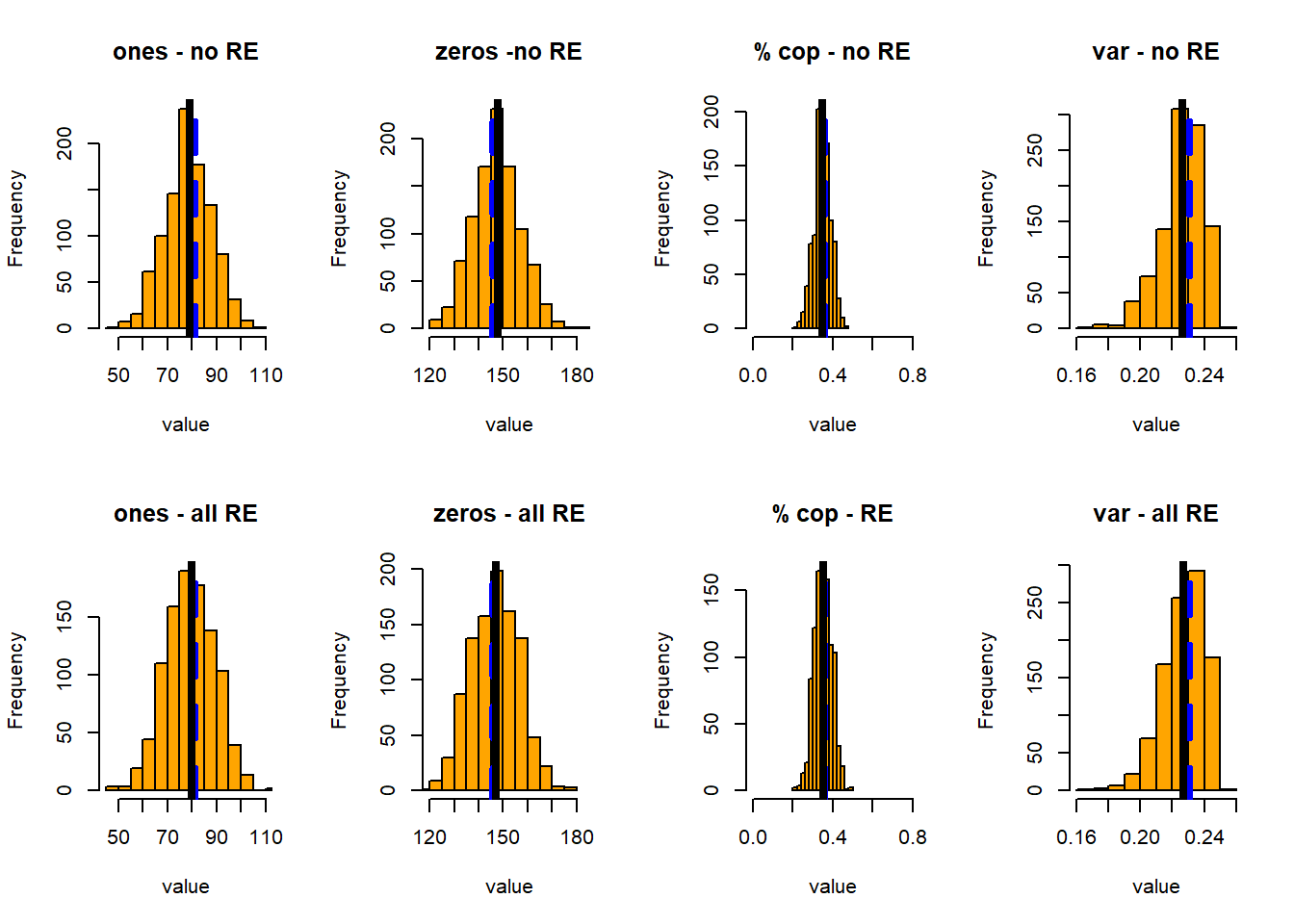par(op)

In this case I’ve plotted the simulated distributions of the number of ones, zeros, the proportion of copulations, and the variance in observed copulations – all with and without accounting for all the random effects. For each of these, I show the distribution of the statistic, its mean (black line), and the mean of the same statistic in the observed data.

All test quantities have broader distributions when we account for the random effects.

## glmmADMB version (not run)

You can run many of the same graphical procedures (including the quick plots from lsmeans!) on output from other frequentist fitting packages. Try it!

## look at glmmadmb too -- not evaluated
gm1admb <- glmmadmb(cop ~ geodist_scl +  sizediff_scl2, random= ~1 | Series + 1 | Female , data=d2, family='binomial')

geom_line(aes(SizeDiff, plogis(upr)), color='orange', size=1, linetype=2) +`